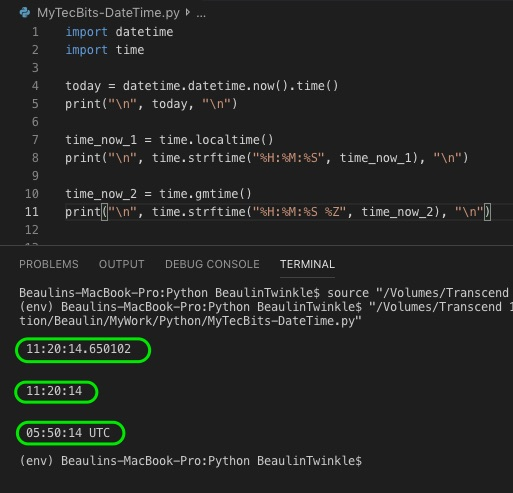# Getting current time alone in python

Earlier we have seen how to get the date part alone from the current system date and time. Now we will see how to get the current time alone from the system date and time. There are several methods to get the current system time. We will see few of them here.

## Method 1: Using datetime module

In this method, we will use the datetime module’s datetime.now() method along with time() to get only the time part form the current date time. Here is an example.

```import datetime

today = datetime.datetime.now().time()
print("\n", today, "\n")
```

## Method 2: Using time module’s localtime method

In this method, we will use the time module’s lacaltime() method. localtime() returns an object struct_time, which is a named tuple. So, we can use the strftime() method to format the data to the required time format. Here is an example.

```import time

time_now = time.localtime()
print("\n", time.strftime("%H:%M:%S", time_now), "\n")
```

## Method 3: Using time module’s gmtime method

In this method, we will use the time module’s gmtime() method. Unlike localtime(), gmtime() returns Greenwich Mean Time or the UTC time in struct_time type, which is a named tuple. So, we can use the strftime() method to format the date to the required time format. This method will be useful when you are working with UTC time. Here is an example.

```import time

time_now = time.gmtime()
print("\n", time.strftime("%H:%M:%S %Z", time_now), "\n")
```

## Reference

### Related Articles & Tools

This site uses Akismet to reduce spam. Learn how your comment data is processed.